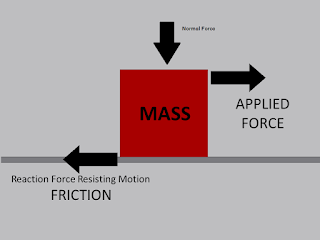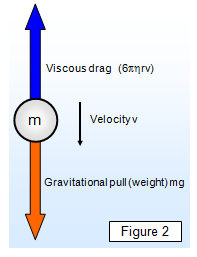## Pages

### 2.3 Friction and viscosity

Here we'll discuss qualitatively the notion of friction and viscosity.

Friction: Friction is the force resisting the relative motion of solid surfaces, fluid layers, and material elements sliding against each other. In other words when two surfaces are in cotact, the forces between them could be represented by a reaction that is normal to the surfaces and a frictional force that is parallel to the surfaces.photocourtesy:curriculum.vexrobotics.com

$$F=\mu N$$
where, $\mu$=coefficient of friction,
$N$=Normal force(weight of an objects)

Viscosity: "Viscous force is the force that resists the relative motion of the body through the fluid."schoolphysics.co.uk
$$F_{v}=6\pi \eta rv$$
where, $\eta$= coefficient of viscosity,
$r$= radius of sphere]
$v$= flow velocity relative to an object

Here's an easy to follow video on viscosity,

Also, here's another video,